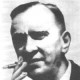# Banach spaces, super-reflexivity, and martingale type

I started reading Convex Games in Banach Spaces, by Sridharan and Tewari and then got interested in the work of G. Pisier on martingales in Banach spaces, which studies how probability and geometry interact. Some of the definitions differ a bit between these papers (the former does things in terms of martingale difference sequences).

A Banach space${\mathcal{B}}$ has Martingale-type${p}$ (or M-type${p}$) if there is a constant${C}$ such that for any${T \ge 1}$ and${\mathcal{B}}$-valued martingale${Y_1, Y_2, \ldots}$, we have$\displaystyle \sup_{t} \mathop{\mathbb E}\left[ \left\| Y_t \right\| \right] \le C \left( \sum_{t=1}^{\infty} \mathop{\mathbb E}[ \left\| Y_t - Y_{t-1} \right\| ^p ] \right)^{1/p}.$

A Banach space${\mathcal{B}}$ has Martingale-cotype${q}$ (or M-cotype${q}$) if there is a constant${C}$ such that for any${T \ge 1}$ and${\mathcal{B}}$-valued martingale${Y_1, Y_2, \ldots}$, we have$\displaystyle \left( \sum_{t=1}^{\infty} \mathop{\mathbb E}[ \left\| Y_t - Y_{t-1} \right\|^q ] \right)^{1/q} \le C \sup_{t} \mathop{\mathbb E}\left[ \left\| Y_t \right\| \right].$

So far these are statements about how martingales behave in these spaces, and you can see a little geometry in the${(\sum (\cdot)^p )^{1/p}}$ kind of stuff, but how does this relate to overall geometric properties of the space?

A Banach space${\mathcal{B}}$ is reflexive if it is isomorphic to its double dual (the dual of its dual space). The space${\mathcal{B}}$ is reflexive if and only if its dual${\mathcal{B}^{\ast}}$ is reflexive. A space${\mathcal{C}}$ is finitely representable in${\mathcal{B}}$ if for any${x \in \mathcal{C}}$ and any${\lambda > 1}$ there exists an isomorphism${\phi : \mathcal{C} \rightarrow \mathcal{B}}$ such that$\displaystyle \lambda^{-1} \|x\| \le \|\phi(x)\| \le \lambda{x}$

A Banach space${\mathcal{B}}$ is super-reflexive if no non-reflexive Banach space is finitely representable in${\mathcal{B}}$. A result of James shows that a space is super-reflexive if and only if its dual is super-reflexive. Reflexivity means a space is “nice” and super-reflexivity is a kind of “uniform niceness.”

The norm for a Banach space is uniformly convex if for every${\epsilon > 0}$ there exists a${\delta > 0}$ such that for two unit vectors${x}$ and${y}$,$\displaystyle \|x + y\| > 2 - \delta$

implies$\displaystyle \|x - y\| < \epsilon.$

For example, for${p > 1}$ the spaces${L_p}$ and${\ell_p}$ are uniformly convex. Another way to picture it is that if the midpoint of the chord between${x}$ and${y}$ is close to the surface of the unit ball, then the chord has to be short. Per Enflo showed that if a Banach space${\mathcal{B}}$ is super-reflexive, it has an equivalent norm which is uniformly convex.

It turns out that the M-type is related to the degree of convexity of a space, namely how uniformly convex the norm is (and in turn connected to super-reflexivity). A space is${q}$uniformly convex if$\displaystyle \left\| \frac{x + y}{2} \right\|^q \le \frac{1}{2} \left( \|x\|^q + \|y\|^q \right) - C^{-q} \left\| \frac{x - y}{2} \right\|^q.$

Gilles Pisier made the connection to M-types : a Banach space is super-reflexive if and only if it has M-type${p > 1}$ (or M-cotype${q < \infty}$). Furthermore, a Banach space has M-cotype${q}$ if and only if${\mathcal{B}}$ has a${q}$-uniformly convex norm.

So the big picture is this: super-reflexivity is enough to guarantee a uniformly convex norm, but the M-cotype characterizes the degree of how convex that norm is.

References:

Sridharan, Kartik and Tewari, Ambuj, Convex Games in Banach Spaces, Proceedings of COLT, 2010.

Enflo, Per, Banach spaces which can be given an equivalent uniformly convex norm, Israel Journal of Mathematics 13 (1972), 281-288.

James, Robert C., Super-reflexive Banach spaces., Canadian Journal of Mathematics 24 (1972), 896Ð904.

Pisier, Gilles, Probabilistic Methods in the Geometry of Banach spaces, in G. Letta and M. Pratelli, ed., Probability and Analysis, v.1206 of Lecture Notes in Mathematics, p167–241, Springer, 1986.

Pisier, Gilles, Martingales with values in uniformly convex spaces, Israel Journal of Mathematics 20 (1975), no. 3-4, 326Ð350.

Pisier, Gilles, Martingales in Banach Spaces (in connection with Type and Cotype), IHP Winter Course online lecture notes, 2011.

Lovaglia, A. R., Locally uniformly convex Banach spaces, Trans. Amer. Math. Soc. 78, (1955). 225Ð238.

1.philipbrooker says:
•Anand Sarwate says: